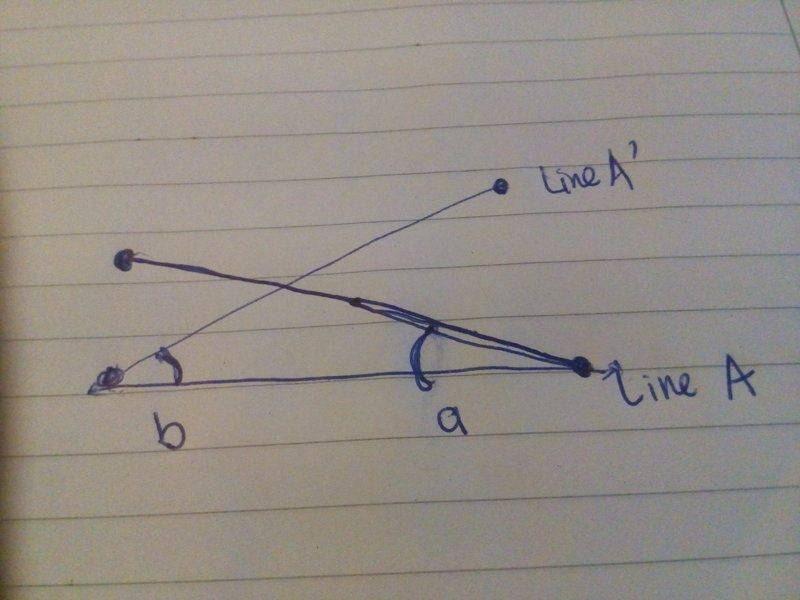# How to calculate angular rotation for a 2D line?

• I
• msn009

#### msn009

For illustration purposes, I have attached an image of the line with the angle that I want to calculate. I am trying to determine the angle of rotation and the calculation that I am using currently is as below:

angle = math.atan2(y,x)

I use this formula to calculate the rotation for A and A', and then I add up the angle of a and b get the rotation. So I am really not sure if this is the correct way to do this. The idea in the end is to see if the rotation from A to A' is >70 degrees or <70 degrees. Sorry my math is pretty rusted at the moment.#### Attachments

Can you explain the problem more, it is not clear at all for me.

line A is the position of the line at timepoint 1 while line A' is the position of the line at timepoint 2. I want to calculate the angle of rotation from the position of the line at timepoint1 to the position of the line at timepoint 2. My approach now is to calculate the angle at each timepoint then I add up these angles to get the degree of rotation. Hope this is clear.

I'm not sure what y and x are, nor what math.atan2 is. (arctan type 2 or arctan squared maybe?)

My feeling would be that each line has a direction - relative to the arbitrary reference frame of the paper - so you can just subtract the initial direction from the final direction to get the rotation.

For eg., if a were 30 deg and b were 25 deg, if you took line ba as East-West, then the starting line dotA would have a bearing of 120 deg and the finishing line bA' would have a bearing of 65 deg giving a rotation of -55 deg.

Your main problems are; finding the angles a and b, and knowing whether a number of complete rotations have occured.

thank you very much Merlin, this was what I was looking for. sorry if the explanation wasn't very clear.

Looking at it now, I realize the bearings could be different, depending on how you label the lines:
Line A is either 120 deg (pointing right) or 300 deg (pointing left)
similarly, line A' is either 65 deg or 245 deg
so the possible rotations are 120 - 65 = 55, 120 - 245 = -125, 300 - 65 =235, or 300 - 245 = 55 degrees.

I think you need to label the ends, eg. A is PQ and A' is P'Q' then you'd be able to see if the line had turned more than 180 deg.

I wonder how you know the angles a and b ?

nor what math.atan2 is. (arctan type 2 or arctan squared maybe?)
atan2 has nothing to do with squaring. atan and atan2 are functions common to many programming languages such as C, C++, C#, python, matlab, and others. Both functions return an angle in radians. atan() takes a single argument, and atan2() takes two arguments.

For example, atan(1.0) returns the numeric equivalent of ##\pi/4##, and atan2(2.0, 1.0) returns about 1.107, which is about 63.4°.